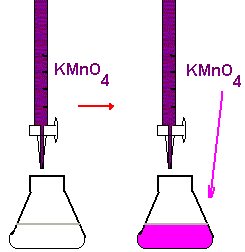Redox Titrations. Potassium Permanganate.
I. Introduction.
 In this experiment you will use a standard solution of potassium permanganate (KMnO4) to determine the  of iron (as Fe2+) in an unknown solution. Permanganate ion reduces to a manganese(II) ion in the acidic solution. This reaction requires 5 electrons and 8 (!) hydrogen ions: MnO4-+  8H+ + 5 e- ® Mn2+ + 4H2O Only one electron is necessary to reduce Fe(III) to Fe(II) Fe3+ + e- ® Fe2+ Therefore, 1 mole of MnO4-(the oxidizing agent) reacts with 5 moles of Fe2+ (the reducing agent) to form 5 moles of Fe3+ and 1 mole of Mn2+. Thus, in net ionic form: MnO4- + 5Fe2+ + 8H+ ® 5Fe3+ + Mn2+ + 4H2O  The 1:5 mole ratio with respect to the amounts of MnO4-and Fe2+ consumed will provide the stoichiometric basis for all of the calculations in this experiment. II. Experimental Procedure. A. Preparation of a Solution of KMnO4 Note: This solution will be prepared in the stockroom and delivered to the students. B. Titration of unknown Fe(II) solution You receive a solution of unknown concentration in 100 mL volumetric flask. Dilute it carefully to the mark. 1. Using a 10 mL pipet, transfer exactly 10.00 mL of an unknown solution into an Erlenmeyer flask. 2. Using a graduated cylinder, add 10 mL of 1 M H2SO4 to the flask.  3. Fill your buret with the KMnO4 solution and drain out enough so that the liquid level is just below the upper calibration mark and the buret tip is full. Read the initial volume from the calibration scale on the buret. This reading and all other buret readings should be estimated to the nearest 0.01 mL. The color of potassium permanganate is so deep that you hardly can see the lower menisk. Use the upper one to read the volumes. 4. Titrate the iron solution in the flask. The pinkish color produced by the first drop of excess KMnO4 signals the end point for the titration. Obtain the final volume reading from the calibration scale on the buret.Note: The color of MnO4 - ion is so bright that NO indicator is necessary with permanganate titrations. 5. Repeat step 4 twice. The volume of KMnO4 solution used should agree with the first titration within 0.20 mL.  III. Calculations. In all calculations we presume that 5 moles Fe(NH4)2(SO4)2 are equivalent to 1 mole KMnO4. 1. Knowing the molarity of your KMnO4 and the volume used in each titration, you can calculate the molarity of your Fe(II) solution as                   molarity(Fe(II)) =  5x molarity(MnO4)´volume(MnO4)/volume(Fe(II)) 2. Knowing the molarity of your Fe(II) solution then allows you to calculate the number of moles of iron (as Fe2+) in your unknown sample and the mass of Fe in g: mass(Fe) = (moles Fe) ´ (55.85 g Fe/mole Fe)            m(Fe)=55.85 x (total volume) x molarity(Fe(II))Take into account that your total volume is 100 mL and atomic weight of Fe is 55.85. 5. Repeat the foregoing calculations for each sample titrated and determine an average value and standard deviation.
Permanganate Titrations.
Name:_________________________Date:___________________
Determination of Iron Unknown.
Molarity of potassium permanganate: _______ M
Volume of the pipet used (volume (Fe): ______mL
Titration 1 __________________mL
Titration 2 __________________mL
Titration 3 __________________mL
mass Fe
#1 __________ # 2 ____________  # 3_____________

Average m (Fe)________________Standard deviation_______________